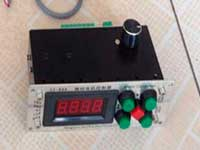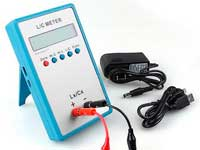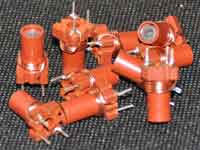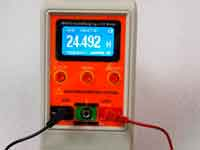Rating 0.00 (0 Votes)

# One turn loop coil

The inductance in the form of an isosceles triangle loop is calculated by the formula:where:

• L - inductance [H];
• a, b - the sides of the triangle [m];
• r - radius of the wire (d/2) [m];
• μ0 = 4π·10-7 permeability of free space;
• μ - relative magnetic permeability of the conductor;

The inductance in the form of a rectangle loop is calculated by the formula:where:

• L - inductance [H];
• a, b - the sides of the rectangle [m];
• r - radius of the wire (d/2) [m];
• μ0 = 4π·10-7 permeability of free space;
• μ - relative magnetic permeability of the conductor;

The inductance in the form of a round loop is calculated by the formula:where:

• L - inductance [H];
• D - diameter of the loop [m];
• d - diameter of the wire [m];
• μ0 = 4π·10-7 permeability of free space;
• μ - relative magnetic permeability of the conductor;

You can often find the last formula in which the last member of the equation is 1.75 instead 2. That variant uses Coil32. In this case, the inductance of the round loop is calculated at high frequency. If the last term is 2, then the inductance is calculated at a low frequency. The low frequency means that the condition is met when the skin depth is greater than the wire radius. At the high frequency the skin depth is much less than the wire radius.

References:

Сomments from anonymous guests are enabled with moderation.

#### Coil Winding Machine###### US \$187.53
The system consists of individual components including a controller, a stepping motor, a foot switch, power adapter and output shaft. This design makes it very easy for you to integrate the system into your own project.

#### LC Meter LC200A###### US \$37.99
Measuring Capacitance Range:
0.01 pF - 100mF
Measuring Inductance Range:
0.001 uH - 100H
Measurement accuracy:1%.

#### RF Inductors###### US \$6.43
Variable Ferrite Core Inductor coil set 3.5Turns 25-100MHZ

#### Auto Ranging LCR Meter###### US \$63.07
Meter can measure 0.00pF to 100.00mF capacitance, 0.000uH to 100.00H inductance and 0.00? to 10.000M? resistance. Very suitable for small value capacitor and inductor measurement.